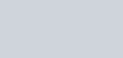Differentiate each of the following w.r.t
Question:

Differentiate each of the following w.r.t $x$ :

$\operatorname{cosec}^{-1}\left(\frac{1+x^{2}}{2 x}\right)$

Solution: